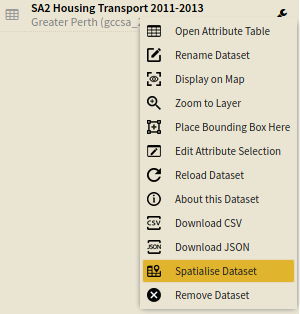# Distance Decay Spatial Weight Matrix

There are a number of methods that have been developed to determine the closeness or nearness between locations.

Among some of the more sophisticated calculate are the distance decay spatial weights matrices. These methods are based on the concept that areas that closer to your area of interest have more of an influence, or are more similar to them than those further away, and thus are weighted as such. This is a more nuanced approach than the Contiguous Spatial Weight Matrix or the Distance Spatial Weight Matrix in that, rather than counting all bordering areas, or all areas within $d$ distance as the same in their ‘closeness’ (and those outside of these criteria as the same in their ‘farness’), the influence of an area decays with distance. This concept is illustrated in the figure below, which shows the decrease in the influence of the areas (fading blue) on the central area (red).In AURIN two versions of distance decay spatial weights matrices are implemented. These are not particularly dissimilar from each other in their outcomes, but the differences are illustrated below:

Inverse distance decay

$w_{ij} = \begin{cases} 1/d_{ij}, \text{ if }d_{ij} \leq d_{max} \\ 0, \text{ if }d_{ij} > d_{max} \end{cases}$Exponential distance decay

$w_{ij} = \begin{cases} exp(-\alpha*d_{ij}), \text{ if }d_{ij} \leq d_{max} \\ 0, \text{ if }d_{ij} > d_{max} \end{cases}$### SET UP

To generate a distance decay spatial weights matrix, we will run the tool on a dataset of for the Darwin GCCSA. To do this:

• Select Greater Perth as your area.
• Select SA2 Housing Transport 2011-2013 as your dataset, selecting all variables.
• Spatialise the dataset that you have just created using the Spatialise Dataset tool in the spanner menu of the dataset.### Inputs

Once you have selected added and spatialised your dataset, open the Distance Decay Spatial Weights Matrix tool (Tools → Spatial Weight Matrices → Distance Decay) and enter the following parameters:

• Dataset Input: The dataset that you would like to calculate the spatial weights matrix. This must contain a geometry column for this tool to be run. Select the spatialised dataset which we generated above.
• Key Column: The column that provides a unique identifier for each row. Select Statistical Area Level 2 Code.
• Type: The type of distance decay model that will be used, inverse-distance or exponential-distance-decay. Select exponential-distance-decay.
• Threshold distance: This specifies the threshold distance in kilometres, $d_{max}$, beyond which areas will have a $w$ value of zero. If you want all of the areas to be included, make sure that the distance you select is larger than the largest distance between areas in the dataset. Type 5.
• Exponential decay parameter: This specifies the value of $\alpha$ in the exponential distance decay equation $exp(-\alpha*d_{ij})$. This value should normally be somewhere between 0 and 1. Type 0.5.
• Style:  This specifies whether the standardisation will be binary, row-standardised, absolute, or globally-standardised. Select row.standardised.
• Matrix Island Parameter:  This specifies how the matrix will deal with islands, which naturally have no contiguous neighbours. Tick this box.Once you have entered these parameters click Run Tool.

### Outputs

Once you have executed the tool, a pop-up will display. Click Display Output and you should see your new dataset look similar to the image below.Zero scores within a cell indicate that the areas are not classified as “close”, and therefore do not affect each other. If a value is greater than zero, it indicates that the area specified by the column influences the area of the row by that much.

This spatial weights matrix can now be used for additional spatial statistical analysis, such as Moran’s I or Geary’s C.

O’Sullivan, D., & Unwin, D. J. (2010). Geographic information analysis and spatial data. Geographic Information Analysis, 1–32.

### Looking for Spatial Data?

You can browse the AURIN Data Discovery:### How can you Create Impact?